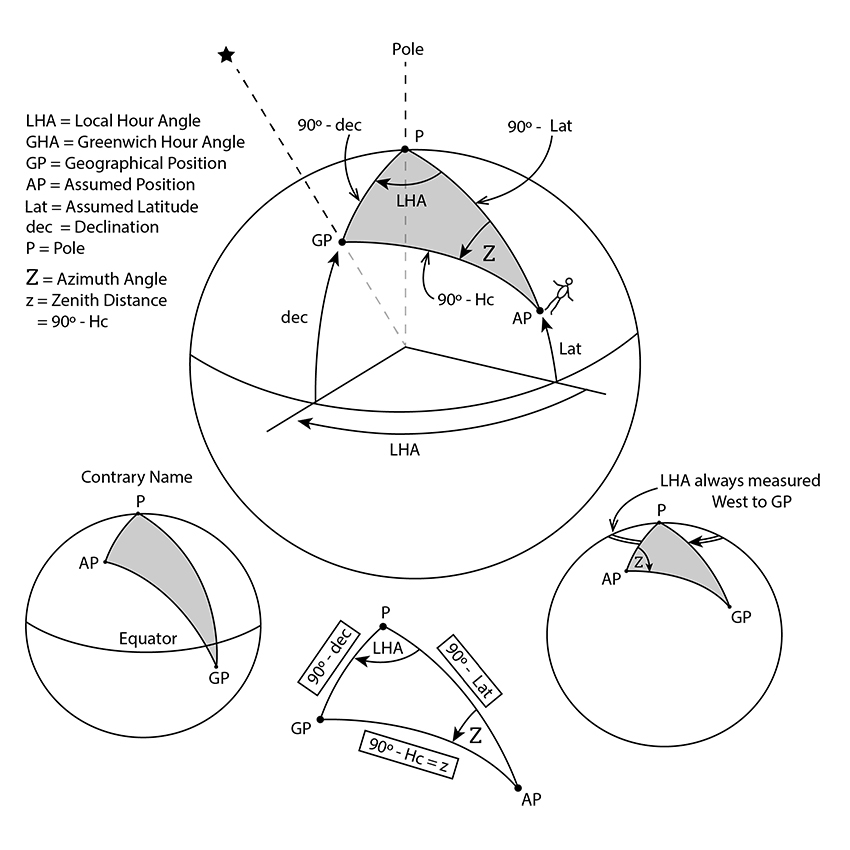|| Starpath online classroom || Celestial Navigation Glossary || Glossary Index || Home || A  B  C  D  E  F  G  H  I  J  K  L  M  N  O  P  Q  R  S  T  U  V  W  X  Y  Z
 Navigational TriangleThe sight reduction method we use in celestial navigation is based on this triangle drawn on the earth's surface. You do not, however, need to know anything at all about this triangle to do celestial navigation and do it well. The corners of the triangle are the north or south pole (P), the assumed position (AP), and the geographical position (GP). The sides of the triangle are: P-AP = (90° - Lat); P-GP = (90° - dec); and AP-GP = (90° - Hc), which is the zenith distance. The angles between the sides that we care about are: Local hour angle (LHA) = the angle between P-AP and P-GP The azimuth angle (Z) = the angle between AP-P and AP-GP The side we care about is the zenith distance (z) which is the length of the line from AP to GP. For any celestial sight, we know two sides and the included angle: (side, angle, side) figured from (dec, LHA, Lat), and from this we can mathematically figure the rest of the triangle. The parts that are solved for in a sight reduction by tables or calculator are Hc and Z. The formulas are: Sin(Hc) = Sin(dec) x Sin(Lat) + Cos(dec) x Cos(Lat) x Cos(LHA) SIGN RULES: (1) All angles are treated as positive, regardless of hemisphere, except for CONTRARY NAME, make DECLINATION A NEGATIVE angle, regardless of hemisphere. and Cos(Z) = [Sin(dec) - Sin(Lat) x Sin(Hc)] / [Cos(Lat) x Cos(Hc)]. SIGN RULES: (1) All angles are treated as positive, regardless of hemisphere, except for CONTRARY NAME, make DECLINATION a NEGATIVE angle, regardless of hemisphere. (2) If the final Z is NEGATIVE, THEN Z = 180 + Z, regardless of hemisphere. ----------------- The formula is for Z (azimuth angle) and not Zn (azimuth). The normal rules for converting Z to Zn based on LHA and Lat apply. Note also, we have two Z's. Upper case Z = azimuth angle; lower case z = zenith distance. This is traditional, so we do not change it.Above notes and graphics adapted from Celestial Navigation: A Complete Home Study Course, Second Edition.